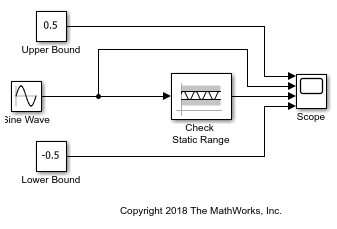# Check Signal Range with Check Static Range Block

Using the Check Static Range block, you can check if an input signal falls outside of an unchanging range of values.In this example, the Check Static Range block compares the value of a Sine Wave block to the values entered in the Upper bound and Lower bound parameters, which are `0.5` and `-0.5`. The Constant blocks, labeled Upper Bound and Lower Bound, illustrate these parameters in the Scope block but do not affect the parameter values. The Check Static Range block checks if the value of the input signal is greater than the Lower bound value or less than the Upper bound value. If it is, the block asserts `true (1)`. Because the Output assertion signal parameter of the block is selected, the block outputs the assertion value. Run the simulation to observe the model output.At the start of the simulation, the Check Static Range block outputs `1` because the value of the Sine Wave block is less than the Upper bound value. At a time of `0.52`, the sine wave exceeds `0.5`. The Check Static Range block recognizes this change and outputs `0`. When the time reaches `2.62`, the sine wave falls below the Upper bound value and the assertion is `true`. When the time reaches `3.67`, the sine wave falls below the Lower bound value. The Check Static Range block recognizes this change and outputs `0`. The output stays at `0` until the time is `5.76`, when the sine wave then exceeds the Lower bound value. This pattern repeats until the simulation end time.